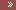THE WORLD OF YOUR POSSIBILITIES - Chapter 3HomePresentationsDATA STRUCTURE NOTES=> Chapter 1=> Chapter 2=> Chapter 3DBMSContactGuestbookLoginToplistCounterTitle of your new pagejhg

Chapter 3: Recursion

3.10    Removal of recursion

Recursion is a name given to a technique for defining a function in terms of itself. A function definition which defines it in terms of a simpler case of itself is called a recursive definition. A definition which defines an object in terms of a simpler case of itself is called a recursive definition.

In order for a function definition not to be circular, the function definition must have the following two properties:-

(i)                     there must be certain arguments, called base values, for which  the function does not refer to itself.

(ii)                Each time the function does refer to itself, the argument of the function must be closer to the base value.

Example- Recursive definition of Factorial Function:-

(i)                     if n=0, then n!=1

(ii)                if n> 0, then, n!=n x (n-1)!

We see that this definition of n! is recursive, since it refers to itself when it uses ( n - 1 )!. In (i), the value of n! is explicitly given when n=0, thus 0 is the base value. In (ii), the value of n! for   arbitrary n is defined in terms of a smaller value of n which is closer to the base value 0. Accordingly the definition of factorial function is not circular, in other words, the function is well-defined.

(1)         4!=4 x 3!

(2)                3!= 3 x 2!

(3)                        2!= 2 x 1!

(4)                                1!=1 x 0!

(5)                                       0!=1

Each case is reduced to a simpler case until we reach the case of 0! which is defined directly as 1. We, backtrack from line (5) to line (1) , returning the value completed in one line to evaluate the result of the previous line. This produces

(5)  0! = 1

(4)  1! =1 x 0! = 1 x 1 = 1

(3)  2! = 2 x 1! = 2 x 1 = 2

(2)  3! = 3 x 2! = 3 x 2 = 6

(1)  4! = 4 x 3! = 4 x 6 = 24

Let us convert this concept of factorial function into C-language function:-

int fact(int n)

{

If ( n == 0)

return 1;

Else

return n * fact(n-1);

}

The sequence of recursive calls when fact(4) is called is as follows:-

 n=4
 n=3
 n=2
 n=1
 n=0
 1
 1
 2
 6
 fact(4)=4 * fact(3)
 fact(3)=3 * fact(2)
 fact(2)=2 * fact(1)
 fact(1)=1 * fact(0)

Now let us write C-program for finding the factorial of a given integer:-

#include<stdio.h>

int fact(int n)

{

if ( n == 0)

return 1;

else

return n * fact(n-1);

}

void main()

{

int x, f;

printf(“n Enter a positive integer:”);

scanf(“%d”,&x);

f=fact(x);

printf(“n The factorial of %d is %d”,x,f);

}

The output of this program is given below:-

Output

Enter a positive integer: 4

The factorial of 4 is 24

Recursive definition of Fibonacci series

(i)                     if n = 0 or n = 1, then fn=n

(ii)                if n > 1, then fn=fn - 2 + fn - 1

This definition of Fibonacci series is recursive, since the definition refers to itself when it uses fn-2 and fn-1.In (i), the base values are 0 and 1 and in (ii), the value of fn is defined in terms of smaller values of n which are closer to the base values. Accordingly this function is not circular, i.e. well-defined.

Let us write C-function for the Fibonacci series:-

unsigned Fib(unsigned n)

{

if(n==0)

return 0;

else if ( n==1)

return 1;

else

return Fib( n-2) + Fib(n-1);

}

The sequence of recursive calls when fib(4) is calls is given below:-

 n = 4
 n = 3
 n = 2
 n = 2
 n = 1
 n = 1
 n = 0
 n = 1
 n = 0
 fib(4)
 fib(3)
 fib(2)
 fib(2)
 fib(1)
 fib(1)
 fib(0)
 fib(0)
 fib(1)

Let us write the C-program for Fibonacci sequence

#include<stdio.h>

unsigned Fib(unsigned n)

{

if(n==0)

return 0;

else if ( n==1)

return 1;

else

return Fib( n-2) + Fib(n-1);

}

void main()

{

unsigned n,i;

printf(“n How many Fibonacci numbers?”);

scanf(“%d”,&n);